# Optimize Hyperparameters with GridSearch

In this blog, we are going to walk through the basics of what hyperparameters are, how they are connected with Grid searching, and then walk through an example notebook that uses Gridsearching to optimize our model.

# What is a Hyperparameter?

A hyperparameter is a parameter whose value cannot be determined from data. The value of a hyperparameter must be set before a model undergoes its learning process. For example, in a RandomForestClassifier model, some of the hyperparameters include: n_estimators, criterion, max_depth, mn_samples_split, etc. (For a full list of the parameters, visit Sci-kit Learn’s RandomForestClassifier model page here).

For the purpose of this blog, we will not be going into the details of each hyperparameter. Hyperparameters are important because they directly control the behavior of the training algorithm and have a significant impact on the performance of the model being trained. Each hyperparameter can take in different amounts of values. For example, n_estimators can take in any integer and criterion can take in either “gini” or “entropy” only. The question that remains is how do we choose the best hyperparameters for our model to produce the best results?

# Enter GridSearch

Grid search is a tool that builds a model for every combination of hyperparameters we specify and evaluates each model to see which combination of hyperparameters creates the optimal model. Instead of us having to manually choose the parameters, we can provide a dictionary where the key is the hyperparameter name and the value is a list of the values we want to try out for that parameter. So let’s say we want to figure out the optimal number for n_estimators and the best value for criterion — it would look something like this:

`# exampleparam_grid = {'n_estimators': [10, 20, 50, 100],'criterion': ['gini', 'entropy']} `

The param_grid dictionary would contain every hyperparameter we would want to tweak for the model, along with a list of different inputs for that hyperparameter.

# Setting Up

The dataset we will be using in this blog will be Sci-kit Learn’s breast cancer dataset. If you would like to follow along in the notebook, you can find it here! First, we will import the necessary libraries:

`# Importing first librariesimport pandas as pdfrom sklearn.datasets import load_breast_cancer`

Next we set up the data:

`# Setting the variable 'dataset' to hold the Bunch object# (special kind of dictionary)dataset = load_breast_cancer()# Creating variables to reference the target and feature columnsfeatures = dataset.datatarget = dataset.target`

If we want to explore the data more, we can create a dataframe to hold the features and target!

`# Creating a dataframe to hold the feature columnsdf = pd.DataFrame(features)# Accessing the Bunch object (dataset) to provide feature names for # the feature columns in our dataframedf.columns = dataset.feature_names# Adding the target to our dataframe by creating the 'target' columndf['target'] = target`

From here we can explore however much we’d like! Let’s go ahead and view the first 5 rows of the dataframe:

`df.head()`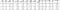Viewing first 5 rows
`df.isna().sum()`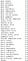Making sure there are no missing values in the data

We are going to quickly swap the classes so that the malignant class is 1 and the benign class is 0. We can do this by creating a small function and then applying the function to the target column of the dataframe. Note that we do not need to do this, I just prefer the malignant class to be set as 1.

`def convert_class(num):   return abs(num — 1)`

Now we simply apply the function to the dataframe:

`converted_target = df[‘target’].apply(convert_class)df['target'] = converted_target`

Now let’s go ahead and view the class distribution:

`# Setting labels for plotlabels ='Benign=0   |   ' + 'Malignant=1'# Viewing number of patients within each classsns.countplot(x=df[‘target’])plt.title(‘Class Distribution’)plt.xlabel(labels);`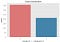Viewing the class distribution

# RandomForestClassifier Information

Since we are using the RandomForestClassifier model in this blog, let’s go over some basic details of the model:

1. RandomForest models have no assumptions
2. Numerical data does not need to be scaled
3. Missing data can affect the Sci-kit Learn model
4. Robust to outliers

Unlike some other models, like linear and logistic regression, random forest models do not have any assumptions about the data. This means that we do not need to worry about things like multicolinearity between features or if residuals are normally distributed. Data used to train random forest models does not need to be scaled, however it does not affect the model negatively if the data is scaled. Because we are going to be using the RandomForest Classifier from Sci-kit Learn, we need to make sure there is no missing data in the dataset. Note that some other versions of RandomForest models do not need any prior handling of missing data. This model is robust to outliers, so we do not need to worry about locating and removing any outliers.

## Some Disadvantages of RandomForest

• Do not predict a continuous output (for regression)
• Does not predict beyond the range of the values in the train set
• Biased towards categorical variables with several categories
• Biased in multiclass problems toward more frequent classes

Since we are focused on Binary Classification and working with strictly numerical data in our features, we do not need to worry about the disadvantages of RandomForest. Here are some advantages.

## Advantages of RandomForest

• Interpretability
• Render feature importance
• Less data pre-processing required
• Do not overfit (in theory)
• Good performance /accuracy
• Robust to noise
• Little if any parameter tuning required
• Apt for almost any machine learning problem

If you have any further questions about RandomForests, feel free to ask me questions in the comments down below or send me an email! For now, we are going to move on to the main course of the blog.

# Creating Train and Test Sets

This is an important step, we must always make sure to create at least a train and test set — otherwise, how would we know if our model is overfitting to the data? Let’s start by importing Sci-kit Learn’s train_test_split:

`from sklearn.model_selection import train_test_split`

Now we can feed in our features and target to create the train and test sets:

`X = df.drop(columns=‘target’)y = df[‘target’]X_train, X_test, y_train, y_test = train_test_split(X, y, random_state=42)`

# Baseline Model

The purpose of the baseline model is to, you guessed it, set the baseline performance! It’s essentially stating the accuracy if one were to blindly guess the class. Our reasoning to create and evaluate a baseline model is to determine how much our actual model has improved when compared to the baseline model. To set this up, we need to import a few things:

`from sklearn.dummy import DummyClassifierfrom sklearn.metrics import plot_confusion_matrix, classification_report`

Now let’s create the DummyClassifier model:

`# Creating and fitting our dummy classifier to train setdummy = DummyClassifier(strategy='stratified')dummy.fit(X_train, y_train)# Creating our y_pred variable off of test featuresy_pred = dummy.predict(X_test)# Printing out the classification reportprint(classification_report(y_test, y_pred))`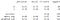Classification report for DummyClassifier

Keep in mind that Class 0 is Benign and Class 1 is Malignant. Since we are working with a dataset that involves cancer, accuracy is not the most important score. We want to make sure that we do not tell someone they do not have cancer when, in fact, they do. Due to the fact that we want an extremely low false negative rate, our focus is on the recall score for the malignant class. Here, we see that the DummyClassifier had a recall score of 24% for malignant class. Let’s see if we can improve that!

# Make Class Weights Dictionary

Because our classes are unbalanced, we can make a class weights dictionary to feed into the model to help the model further differentiate between the classes by punishing the model harder for incorrect guesses. We can set this up by importing:

`from sklearn.utils import class_weight`

Now that we have imported Sci-kit Learn’s class_weight, we can now create a dictionary to hold our class weights!

`# A array object that contains the weights for both classesclass_weights = class_weight.compute_class_weight(‘balanced’, classes=np.unique(y_train), y=y_train)# A dictionary object containing key-value pairs of both classes and # their weightsclass_weights_dict = {0: class_weights, 1: class_weights}print(f”Our class weights:\n{class_weights_dict}”)`

# Instantiating A RandomForestClassifier

We are almost to the GridSearch — all we need to do now is instantiate our model, create a hyperparameter grid that contains ranges of values for each hyperparameter we want to explore, and then fit it to a GridSearchCV object. Let’s begin by creating our model:

`# Importing necessary library to create our modelfrom sklearn.ensemble import RandomForestClassifier# Creating our model and passing in the class weightsforest = RandomForestClassifier(class_weight=class_weights_dict)`

# Hyperparameter Grid

Now let’s create our grid! This grid will be a dictionary, where the keys are the names of the hyperparameters we want to focus on, and the values will be lists containing different values that specific hyperparameter can accept.

`# Creating a dictionary called params to hold our gridparams = {‘n_estimators’: [10, 25, 50, 100, 200], ‘criterion’: [‘gini’, ‘entropy’], ‘max_depth’: [3, 5, 10, 15, 20, 25, None], ‘min_samples_leaf’: [1, 2, 5, 10]}`

An important note: be aware of how many different hyperparameters you choose to evaluate in the gridsearch, along with the number of values in the lists. One downside to Gridsearching is the amount of time it can take to run… if we had a big dataset, this would take an extremely long time (I have had gridsearches run for over 8 hours before).

# Setting Up the GridSearchCV

Now that we have created a model and a grid, we can finally create a GridSearchCV object and fit it to our training data! First let’s import the necessary libraries:

`from sklearn.model_selection import GridSearchCV`

Next, we will make an instance of the GridSearchCV:

`clf = GridSearchCV(estimator=forest, param_grid=params, scoring=’recall’, cv=5)`

Notice above, we provide the estimator with our model, the param_grid with our hyperparameter grid, have selected the scoring to focus on recall, and left the cv as the default integer of 5. CV is cross-validation, which determines the number of folds in the cross-validation splitting strategy.

Now let’s fit the GridSearchCV object to our training set:

`# fitting clf to train setclf.fit(X_train, y_train)`

Note that this can take a considerable amount of time depending on the number of parameters, number of values to try for each parameter, and the amount of data we are using. Once the fitting is complete, we can view the best parameters by calling:

`best_params = clf.best_params_print(best_params)`

Now that we’ve figured out the optimal values for the selected hyperparameters based on the values we’ve provided, we will create a new random forest model that will use these hyperparameter values. We do not have to manually type in the optimal values for each parameter based off of our Gridsearch. Instead, we can easily unpack the best_params dictionary into the new model by putting two asterisks before best_params like so:

`# unpacking the best_params into our new modelbest_forest = RandomForestClassifier(**best_params, class_weight=class_weights_dict)`

Now that we’ve created our model with the optimal parameters, let’s fit it to the training data:

`# Fitting our model to the train setfit_forest = best_forest.fit(X_train, y_train)# Creating predicted variables to compare against y_testy_pred = fit_forest.predict(X_test)# making classification report and confusion matrixprint(classification_report(y_test, y_pred))plot_confusion_matrix(fit_forest, X_test, y_test, normalize=’true’, cmap=’Reds’)`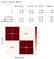Classification Report and Confusion Matrix for Optimal Model

In a nutshell, that is a basic way of how to gridsearch a model’s hyperparameters to find the best values for each specified parameter within the grid. Remember that the more keys, values, and data you have when gridsearching, the longer it will take for gridsearch to complete the exhaustive search. In this blog we performed a basic gridsearch on only a few hyperparameters of the RandomForestClassifier. A link to the notebook can be found here. I hope you enjoyed, feel free to reach out if you have any questions!

## Analytics Vidhya

Analytics Vidhya is a community of Analytics and Data…

## Analytics Vidhya

Analytics Vidhya is a community of Analytics and Data Science professionals. We are building the next-gen data science ecosystem https://www.analyticsvidhya.com

Written by

## Christopher Lewis

I am an aspiring Data Scientist and Data Analyst skilled in Python, SQL, Tableau, Computer Vision, Deep Learning, and Data Analytics.## Analytics Vidhya

Analytics Vidhya is a community of Analytics and Data Science professionals. We are building the next-gen data science ecosystem https://www.analyticsvidhya.com

## More From Medium

Medium is an open platform where 170 million readers come to find insightful and dynamic thinking. Here, expert and undiscovered voices alike dive into the heart of any topic and bring new ideas to the surface. Learn more

Follow the writers, publications, and topics that matter to you, and you’ll see them on your homepage and in your inbox. Explore

If you have a story to tell, knowledge to share, or a perspective to offer — welcome home. It’s easy and free to post your thinking on any topic. Write on Medium